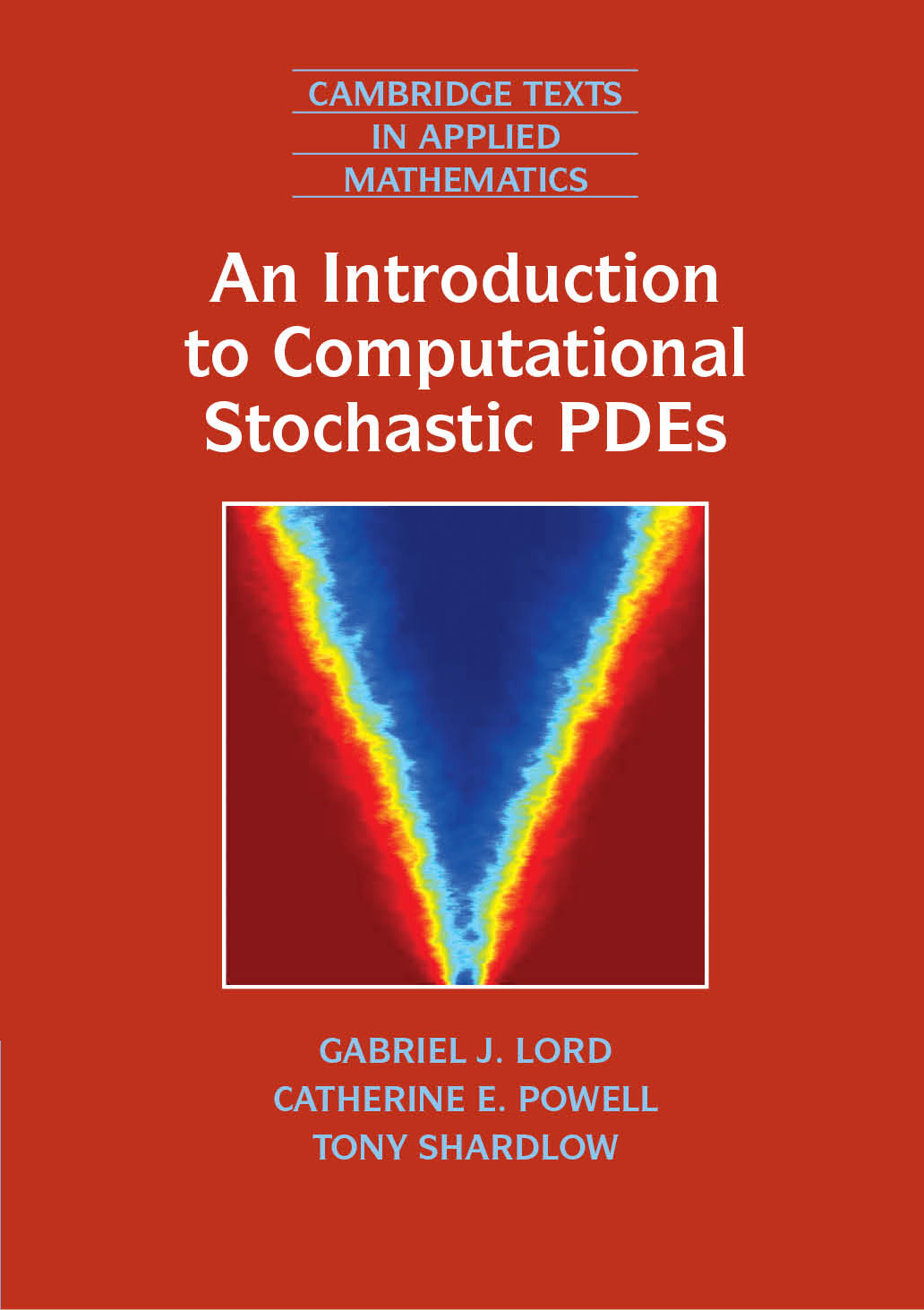An Introduction to Computational Stochastic PDEs

Authors

Catherine E. Powell (Manchester University)
Tony Shardlow (Bath University)

Cambridge Texts in Applied Mathematics, CUP
Hardback (ISBN: 9780521899901)
Paperback (ISBN: 9780521728522)
and eBook.
To order :

 BibTex Entry from MathSciNet Review from MathSciNet

Abstract

This book gives a comprehensive introduction to numerical methods and analysis of stochastic processes, random fields and stochastic differential equations, and offers graduate students and researchers powerful tools for understanding uncertainty quantification for risk analysis. Coverage includes traditional stochastic ODEs with white noise forcing, strong and weak approximation, and the multi-level Monte Carlo method. Later chapters apply the theory of random fields to the numerical solution of elliptic PDEs with correlated random data, discuss the Monte Carlo method, and introduce stochastic Galerkin finite-element methods. Finally, stochastic parabolic PDEs are developed. Assuming little previous exposure to probability and statistics, theory is developed in tandem with state-of the art computational methods through worked examples, exercises, theorems and proofs. The set of MATLAB codes included (and downloadable) allows readers to perform computations themselves and solve the test problems discussed. Practical examples are drawn from finance, mathematical biology, neuroscience, fluid flow modeling and materials science.

Contents

Preface
PART I: Deterministic differential equations PART II: Stochastic processes and random fields PART III: Stochastic differential equations